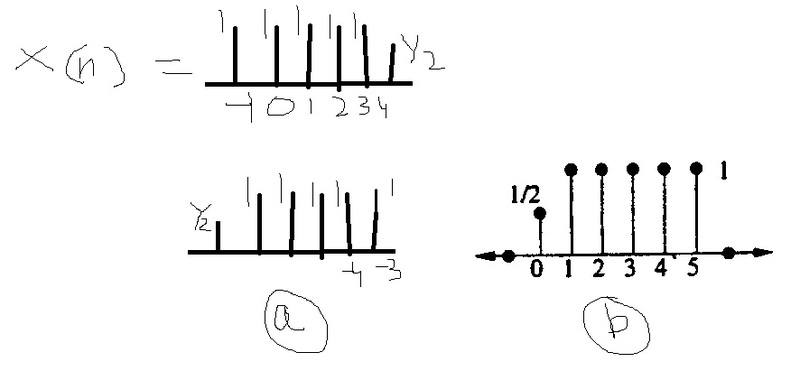# Time Shifting of Signals

coolrpKindly see the question above.

First signal is x[n]

Ques 1: we are required to find x[4-n]

The way I did, the answer should be (a) but one of the solution says (b).

Which is the correct one?

Ques 2: What are we to do while finding x[2n]? Consider only 0, 1 and 2 as twice of those gives 0, 2 and 4 which is what we have from x[n]? or does the whole signal redistribute at -2, 0, 2,4, 6, 8?

Ques 3: How do we calculate x[n]u[2-n]?
I took unit step signal, flipped it at 0 to get u[-n] and then shifted it by 2 which makes the new step signal from -2 to - infinity. Multiplying this with x[n] gives zero. Is it correct?